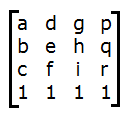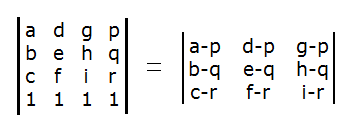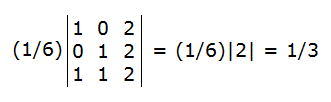# Tetrahedral Volume from Vertex Coordinates

Tetrahedron Volume Calculator
( , , )

( , , )

( , , )

( , , )

In geometry, tetrahedra are 3-dimensional solids that have 4 vertices, 6 edges, and 4 triangular faces. They are also known as "triangular pyramids;" in their natural orientation they have a triangular base and 3 triangular sides that meet at a point.

If you know the coordinates of the vertices of a tetrahedron, you can compute its volume with a matrix formula.

You can also use the convenient volume calculator on the left. Simply enter the coordinates of four vertices in any order and click the "Find Volume" button.

### The Matrix Formula

Call the four vertices of the tetrahedron (a, b, c), (d, e, f), (g, h, i), and (p, q, r). Now create a 4-by-4 matrix in which the coordinate triples form the columns of the matrix, with a row of 1's appended at the bottom:The volume of the tetrahedron is 1/6 times the absolute value of the matrix determinant. For any 4-by-4 matrix that has a row of 1's along the bottom, you can compute the determinant with a simplification formula that reduces the problem to a 3-by-3 matrix:Example: A tetrahedron has vertices at (0, 0, 0), (1, 0, 1), (0, 1, 1), and (2, 2, 2). We can use the simplification formula and compute the determinant of a 3-by-3 matrix whose columns are (1, 0, 1), (0, 1, 1), and (2, 2, 2). Dividing this determinant by 6 yields the volume.In this example, the volume of the tetrahedron is 1/3.